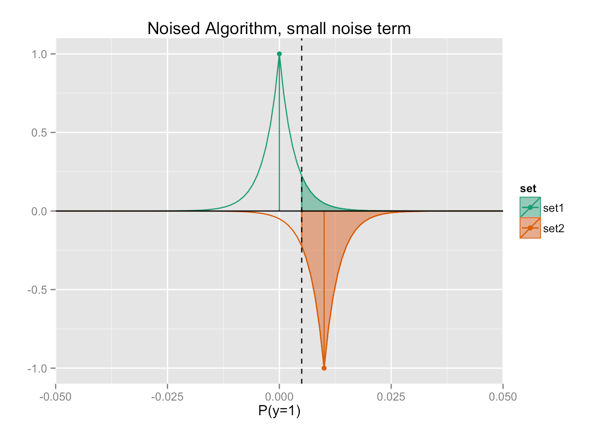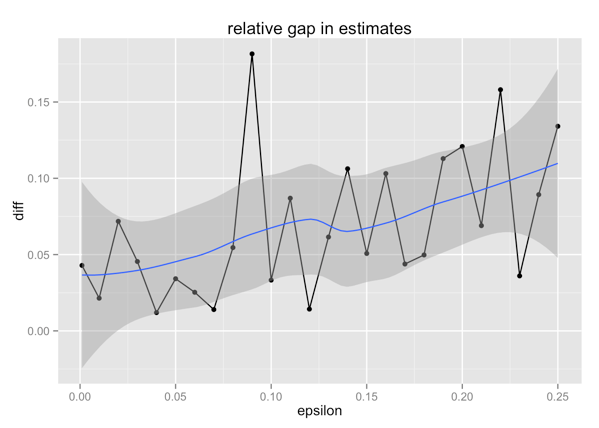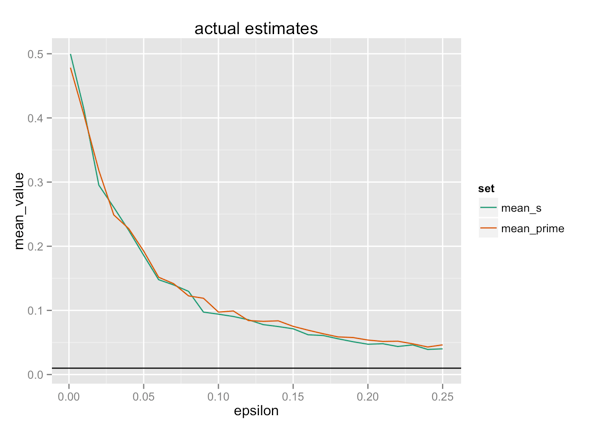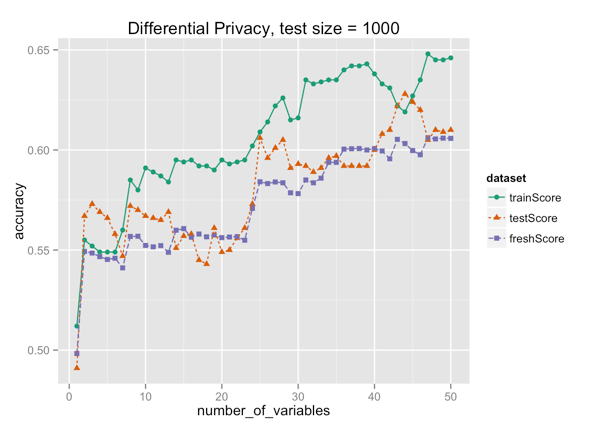# A Simpler Explanation of Differential Privacy

Differential privacy was originally developed to facilitate secure analysis over sensitive data, with mixed success. It’s back in the news again now, with exciting results from Cynthia Dwork, et. al. (see references at the end of the article) that apply results from differential privacy to machine learning.

In this article we’ll work through the definition of differential privacy and demonstrate how Dwork et.al.’s recent results can be used to improve the model fitting process.The Voight-Kampff Test: Looking for a difference. Scene from Blade Runner

## The Definition of Differential Privacy

We can define epsilon-differential privacy through the following game:

• A learner implements a summary statistic called A().
• A (notional) adversary proposes two data sets S and S’ that differ by only one row or example, and a test set Q.
• A() is called epsilon-differentially private iff:

| log( Prob[A(S) in Q] / Prob[A(S’) in Q] ) | ≤ epsilon

for all of the adversary’s choices of S, S’ and Q. The probabilities are defined over coin flips in the implementation of A(), not over the data or the adversary’s choices.

The adversary’s goal is to use A() to tell between S and S’, representing a failure of privacy. The learner wants to extract useful statistics from S and S’ without violating privacy. Identifying a unique row (the one which changed markings) violates privacy. If the adversary can tell which set (S or S’) the learner is working on by the value of A(), then privacy is violated.

Notice S and S’ are “data sets” in the machine learning sense (collections of rows carrying information). Q is a set in the mathematic sense: a subset of the possible values that A() can return. To simplify the demonstration, we will take Q to be an interval.

In the following examples, A() returns the (approximate) expected value of a set s. The adversary has chosen two sets S and S’ of size n = 100:

• S = {0,0,0,…,0} (100 zeros)
• S’ = {1,0,0,…,0} (1 one and 99 zeroes)

The set Q will be an interval [T, 1], where the adversary picks the threshold T.

The adversary’s goal is to pick T such that when he sees that A(s) ≥ T, he knows that A() has just evaluated S’. The learner has two (competing) goals:

• To pick an algorithm A() such that A(S) and A(S’) are so “close” that the adversary can’t pick a reliable T, to preserve differential privacy. “Close” is defined by epsilon.
• To have A() be a good estimate of the expectation, for performing useful analysis.

## The Deterministic Case

Here, A(s) simply returns the expected value `mean(s)`, so it always returns A(S) = 0 when evaluating S, and A(S’) = 0.01 when evaluating S’. This is clearly not differentially private for any value of epsilon. If the adversary picks T = 1/200 = 0.005, then he can reliably identify when A() has evaluated the set S’, every time they play a round of the game.In the figures, set1 in green represents the distribution of values returned by calls to A(S), and set2 in orange returns the distribution of values returned by calls to A(S’). I’ve plotted set2 upside down for clarity.

## Adding Noise to give Privacy

One way for the learner to obscure which set she is evaluating is to add a little random noise to the estimate, to “blur” it. Following Dwork et.al.’s methods, we’ll add noise from a Laplace distribution, which is symmetric and falls off slower than gaussian noise would. Here we show adding just a little bit of noise (of “width” sigma = 1/3n):The shaded green region represents the chance that A(S) will return a value greater than the adversary’s threshold T — in other words, the probability that the adversary will mistake S for S’ in a round of the game. We’ve now made that probability non-zero, but it’s still much more likely that if A(s) > T, then s = S’. We need more noise.

In particular, we need the noise to be bigger than the gap A(S’)-A(S) = 1/n, or 0.01. Let’s pick sigma = 3/n = 0.03:Now we see that the shaded green region has almost the same area as the shaded orange region — you can think of epsilon as expressing the difference between the shaded green region and the shaded orange region. In fact, the absolute value of the logratio of the two areas is epsilon. In other words, observing that A(s) > T is no longer strong evidence that s = S’, and we have achieved differential privacy, to the degree epsilon.

Of course, A(s) is also no longer a precise estimate of `mean(s)`. We’ll return to that point in a bit.

### Simulating the Game

We can simulate the game I described above in R. I’ve put the code on github, here.

The code sweeps through a range of values of epsilon, and for each value selects a suitable noise width and runs 1000 rounds of the differential privacy game, returning a value for A(S) and A(S’). Each round we record whether A(S) and A(S’) are greater than T = 1/200.

## From Differential Privacy to Machine Learning

Differential privacy aims to make the answers to “snooping queries” too vague to distinguish closely related sets (in this case, it makes the probability that A(S) ≥ T about the same as the probability that A(S’) ≥ T). But for machine learning, we are also interested in the output of A(). We can use the simulation above to estimate what happens to A(S) and A(S’) for different values of epsilon. Here we plot the (normalized) gap between the expected values of A(S) and A(S’) as a function of epsilon, from the simulation:As epsilon gets smaller (implying stricter privacy), the relative gap between the expected values of A(S) and A(S’) gets smaller. The discrepancies in the plot are probably due to poor choices of the noise parameter (I picked them heuristically), but the trend is clear. This makes sense, since privacy implies that the A(S) should look a lot like A(S’).

However, as epsilon gets stricter, the estimates of `mean(S) = 0` and `mean(S') = 0.01` — which is what A() is supposed to estimate — also become poorer.This is the trade-off: how can we preserve privacy and still perform useful analysis?

### Differential Privacy and Adaptive Data Analysis

The recent papers by Dwork, et.al. apply differential privacy to the problem of adaptive data analysis, or reusable test sets. In standard machine learning practice, we use two data sets to model: a training set to fit the model, and a test set to evaluate the model. Ideally we only use the test set once; but in practice we go back to it again and again, as we try to improve our model. We already know that performance estimates of a model over its training set are upwardly biased (the model looks more accurate on training than it really is, because it “knows” the training set); if we go back to the test set too many times, then performance estimates of the model on the test set are also upwardly biased, because we also “know” the test set. This problem is exacerbated when we also use the test set to tune the model: for example to pick the variables we use, or tune modeling parameters. In other words, even if we observe the best practice of using a training set to fit models, a calibration set to tune models, and a holdout set to evaluate the model, we can also contaminate the calibration set if we look at it too many times.

Dwork, et.al., describe how (and how many times) we can go back to a test set without biasing its evaluations of model performance. To do this, we make sure that the modeling procedure only interacts with the test set in a differentially private manner. Because it has limited access to the test set, the modeling procedure can’t learn the test set, so evaluations of a model over the test set still accurately reflect a model’s performance on unseen data.

Describing the proofs and techniques in these papers is outside the scope of this article, but we can demonstrate the results in a simple example, similar to the example application shown in Dwork, et.al.’s Science paper.

### Using Differential Privacy for Stepwise Regression

For our example, suppose we have 2000 rows of data with a binary outcome y (50% prevalence of the positive class), and 110 possible input variables to choose from. In our tests we used synthetic data with ten variables (`x1...x10`) that carry signal and one hundred noise variables (`n1...n100`).

We decide to use forward-stepwise logistic regression to choose a suitable model for predicting y. We split the sample data into 1000 rows for the training set, and 1000 rows for the test set. Given that we have selected k-1 variables and have fit a model, M(k-1), that uses those variables, we pick the kth variable by fitting a model Mnew on the training set using the (k-1) previously selected variables plus one new variable from the remaining candidates. We then evaluate the accuracy of M(k-1) and Mnew on the test set, and pick as the kth variable the one for which Mnew showed the most improvement. In other words, we fit candidate models on the training set, and evaluate them for improvement on the test set. This is a fairly common way of tuning models. Standard implementations of stepwise regression often use training set AIC as the criterion for picking the next model; we use accuracy improvement to keep the code straightforward, and closer to the assumptions in the paper.

Normally, the stepwise procedure would terminate when the model stops improving, but for this example we let it run to pick fifty variables. At each step we recorded the accuracy of the selected model on the training set (green circles), the test set (orange triangles) and on a fresh holdout set of 10,000 rows (purple squares). We picked this additional large holdout set (called “fresh”) to get a good estimate of the true accuracy of the model. Here’s the performance of the naive procedure:The test set performance estimates were are more upwardly biased than the training set estimates! This is not too surprising, since we are using the test set to select variables. Despite the optimistic curve of the test scores, we can see from the true out-of-sample performance (`freshScore`) that the model performance is not much better than random; in fact, the algorithm only picked one of the ten signal carrying variables (the first one selected). The test set (and to a lesser extent, the training set) believe that performance continues to improve, when in fact performance degrades as more noise variables swamp out the single signal variable.

We want to use the test set to pick variables, while also using it to accurately estimate out-of-sample error. In their recent Science article, Dwork, et.al. propose an algorithm called Thresholdout to let us safely reuse the test set. We implemented our `diffPrivMethod` based on Thresholdout. `diffPrivMethod` evaluates proposed models on both the training and test sets. If the model’s delta accuracies on the test and the training sets are within a certain tolerance, then `diffPrivMethod` returns the training set delta accuracy with a bit of Laplacian noise as the model score. If the two delta accuracies are far apart, then `diffPrivMethod` adds a bit of Laplacian noise to the test set delta accuracy, and returns that as the model score. In R, the code would look something like this:

```# Return an estimate of model performance in a
# differentially private way
# v is the new candidate variable
# varSet is the set of already selected variables

diffPrivMethod = function(v) {
# Original model was fit on
# training data using varSet;
# Fit a new model using varSet+v
# using the training data
# and evaluate the difference
# between the new models on the test set
testScore <- modelScoreImprovement(varSet,v,
trainData,
testData)
# Do the same thing, but evaluate
# the improvement on the training set
trainScore <- modelScoreImprovement(varSet,v,
trainData,
trainData)

if(abs(testScore-trainScore)<=eps/2) {
# if the scores are close,
# return trainScore with a little noise
sc <- trainScore + rlaplace(1,eps/2)
} else {
# otherwise, return testScore
# with a little more noise
sc <- testScore + rlaplace(1,eps)
}
# make sure the score is in range [0,1]
pmax(0,pmin(1,sc))
}
```

We picked the tolerance and the widths of the Laplace noise somewhat arbitrarily.

The beauty of this scheme is that we never look directly at the test set. If the test and training set performances are similar, we see the (noisy) training performance; when we do look at the test set, we only see a noisy view of it, so it leaks information more slowly.

The original Thresholdout algorithm does not add noise to the training set performance, probably under the reasoning that we know it already. We decided to add noise anyway, on the principle that even knowing when you are using the test set performance leaks a little information, and we wanted to reduce the leakage a little more.

Here are the results of the stepwise regression using `diffPrivMethod` (we set the tolerance/noise parameter eps=0.04 for this run):Now the test set estimates the true out-of-sample accuracies quite well. The chosen models achieve a peak accuracy of about 61%, at around 36 variables. This method did a better job of picking variables — it found all ten signal variables — though it did start picking noise variables fairly early on.

However, there is still money left on the table. What if we used a really large test set? Here, we show the results of running the naive stepwise regression (no differential privacy), with the same training set of 1000 rows and a test set of 10,000 rows.Now the learning algorithm picks nine of the signal variables immediately, and achieves an accuracy of 62%. We can see that the model’s performance on the test set is still upwardly biased, but much less so than with the naive method. Because the test set is larger, we don’t contaminate it as much, even when looking at it directly.

This suggests that we can think of differential privacy as letting us simulate having a larger test set, though obviously we still need our actual test set to be a reasonable size, especially since stepwise regression requires a lot of queries to the test set. Our results also show that while these new differential-privacy based schemes let us do a good job of estimating out-of-sample performance, that alone is not enough to insure best possible model performance.

All code, data, and examples for this experiment can be found here.

## References

Dwork, Cynthia, Vitaly Feldman, Moritz Hardt, Toniann Pitassi, Omer Reingold, Aaron Roth. “Preserving Statistical Validity in Adaptive Data Analysis”, arXiv:1411.2664, April 2015.

Dwork, Cynthia, Vitaly Feldman, Moritz Hardt, Toniann Pitassi, Omer Reingold, Aaron Roth. “The reusable holdout: Preserving validity in adaptive data analysis”, Science, vol 349, no 6248 pp 636-638, August 2015. [link to abstract]

Blum, Avrim and Moritz Hardt. “The Ladder: A Reliable Leaderboard for Machine Learning Competitions”, arXiv:1502.04585, February 2015.### Nina Zumel

Data scientist with Win Vector LLC. I also dance, read ghost stories and folklore, and sometimes blog about it all.

### 6 replies ›

1.Shanx says:

Thanks for such a clear exposition!

The original papers show that the noise parameter is a function of the number of rows in the test set, number of queries, and the form of the statistic. The problem is, one would have to analytically derive the noise parameter for a statistic of choice.

These simulations suggest it might be possible to arrive at the noise parameter for a given dataset and learning algorithm by running the right simulations. Do you think that’s possible?

1.Nina Zumel says:

Thanks for the excellent question! It so happens that for the simulations we ran in this article, we swept over a range of candidate values for a good noise parameter. I hesitate to recommend that as a general practice, because that is going “back to the well” of the dataset yet again.

In this case, we were generating the data, so we had as much of it as we wanted, and in practice I suppose one could have a separate calibration set to find good parameters, or use bootstrap simulation to generate another data set with appropriate distributions. But I haven’t thought too deeply about the implications of bootstrap sampling; my goal in this post was simply to demonstrate the technique.

It’s also worth noting that from our simulations the noise parameters that produce the model with the best *actual* out-of-sample performance are not necessarily the parameters for which the test set best *approximates* out-of-sample error. In other words, the best-performing model can still be upwardly biased with respect to the test set. Thresholdout is designed to optimize getting a good approximation of out-of-sample error, rather than directly optimizing out-of-sample model performance. While the two are related, there are still trade-offs.

2.John Mount says:

It looks like even though the paper isn’t open access the supporting material (and code) are: http://www.sciencemag.org/content/suppl/2015/08/05/349.6248.636.DC1 . Looking through the code we see the science graphs were not generated using Laplace noise as the authors seem to imply in the article, but a randomized chance of normal noise:

``` if abs(ftrain-fholdout) < threshold + np.random.normal(0,tolerance): fholdout = ftrain else: fholdout += np.random.normal(0,tolerance) ```

Though I think the noising of test and training used in your example may have similar benefit as the noised choice threshold (making it more obscure how test exactly relates to training when they are close to the threshold).

I think this very much supports that there is still room for experiment as to what are the best masking procedures (such as using a deterministic switch condition or, Bootstrapping http://www.win-vector.com/blog/2015/10/a-simple-differentially-private-procedure/ ).

And I found a couple more interesting commentaries on the Science article:

Also this is a good place to emphasize your demonstration is stepwise logistic regression (a hard problem of great utility to analysts that needs a lot of sub-queries) and the original paper is essentially demonstrating model size selection from roughly independently scored single variable models (also a good problem, but needs fewer queries as it doesn’t fully model variable to variable dependencies).

Also it looks like the science graphs appear “more stable” because they are actually the aggregate of 100 runs each- not the performance seen in single experiment.

3.john says:

Very good post. It help me a lot to understand the DP algorithm.

4.jpillow says:

What I find puzzling about the Dwork et al paper is that it isn’t clear whether the problem it’s aiming to fix really exists or not. Does anyone use the TEST set error for variable selection? The forward-stepwise regression example shown here shows very clearly how bizarre this would be, since (as Nina points out) it leads to a scenario where test performance goes up much more quickly than training performance (“naive method” figs above).

Has anyone ever done this before? Has anyone seen a published paper using a method that gives smaller test error than training error? That seems fairly ludicrous to me. And as far as I can tell, Dwork et al don’t point to any papers in the literature that are guilty of the crime their method addresses.

[Technical remark: Nina notes that you would normally use AIC to pick the next regressor, but of course the penalty would be the same for all models with K+1 parameters, so really you’re just using training error to select which variable to add. And that’s what leads to the ‘standard’ scenario where training performance rises monotonically as you add regressors, but test performance behaves like an inverted U-shaped function. The REAL question should be: if you use the peak of this U-shaped function to select which model to use and report that peak as your test performance — which I think is standard if not enlightened practice — how biased is your reported test performance?].

Anyway, I don’t mean to be too negative because re-using test sets surely *is* a problem, and the idea of addressing it with differential privacy certainly seems novel and cool. I’m just curious to know how bad of a problem it is likely to be in terms of current practice, and I’m fairly surprised none of the reviewers at Science asked them to show application to a more realistic setting, i.e., where we can see how pernicious the problem is likely to be and how much of an improvement DP really provides.

Thanks Nina for an entertaining and informative post. I found this very clear and much easier to understand than the original paper!

5.John Mount says:

(John here) Thanks for your comment!

Nina and I did try some empirical runs (linked in article) and came to the conclusion that reserving some test data for step variable scoring is usually worse than the standard method of using all our training data to run stepwise regression. Also of interest was: if it was just a matter of picking how many variables (stopping) we wouldn’t have big problem- it is paying the multiple comparison penalty for all the different sets of variables you end up trying.

It is an interesting technique, but also kind of remarkable it got a science article using only one or two synthetic data sets (and also using a series heavily aggregated performance graphs). The acid test would indeed be publicly outperforming some standard technique.